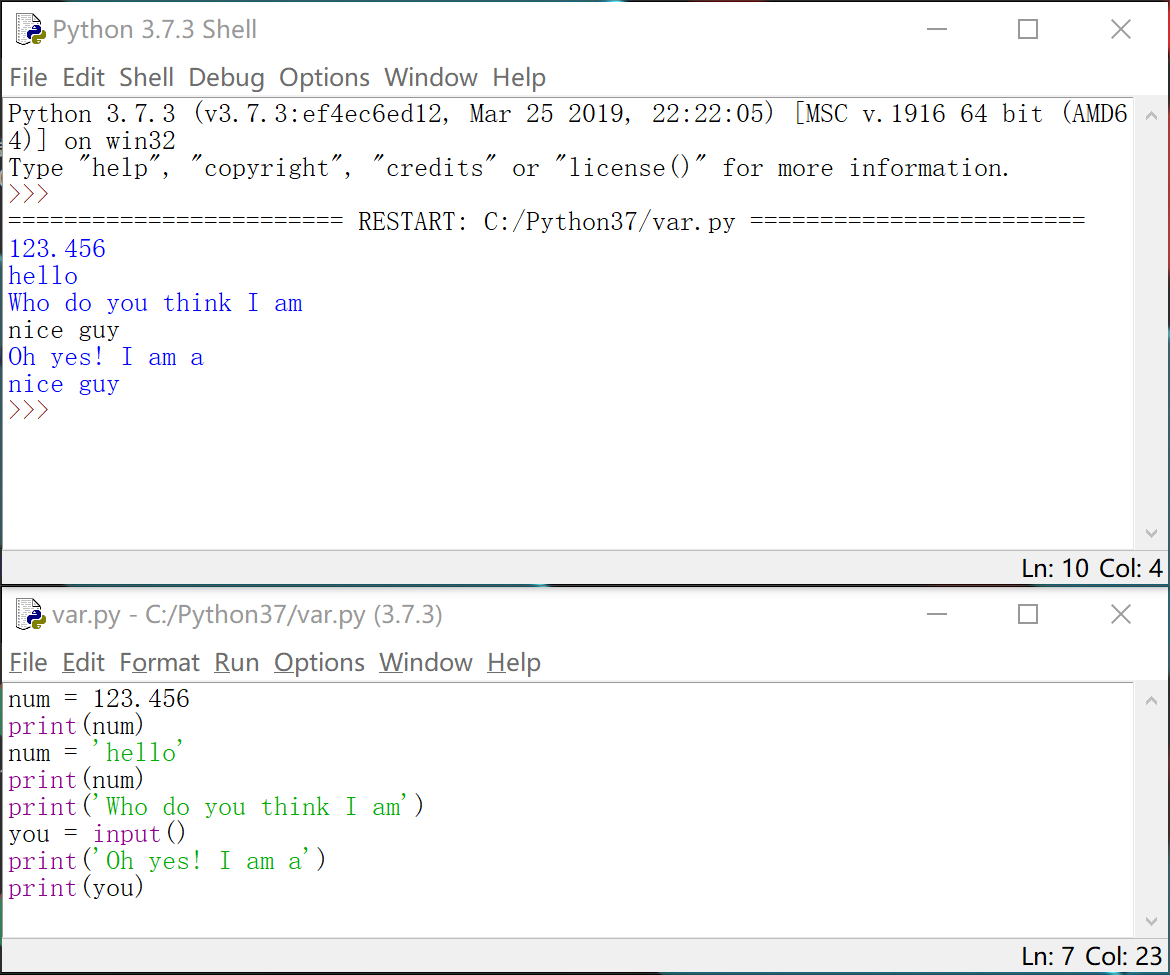## 【Python 第5课】变量

Python之神说，要有变量！于是就有了变量。

``````name = 'Crossin'
myVar = 123
price = 5.99
visible = True``````

=”的作用是把右边的值赋予给左边的变量。

• 字符串 ： 表示一串字符，需要用''单引号或""双引号包围起来
• 整数
• 浮点数 ： 就是小数
• bool（布尔）： 这个比较特殊，是用来表示逻辑上的“真”和“假”（或者说“”和“”）的一种类型，它只有两个值，True False。（注意：这里没有引号，有了引号就变成字符串了）

``````name = 'Crossin'
print(name)``````

``````a = 123
print(a)
a = 'hi'
print(a)``````

“=”的右边还可以更复杂一点，比如是一个计算出的值:

``````value = 3 * 4
print(value)
value = 2 < 5
print(value)``````

``````name = input()
print(name)``````

``````print("Who do you think I am?")
you = input()
print("Oh, yes! I am a")
print(you)``````#======== 课外的话 ========#